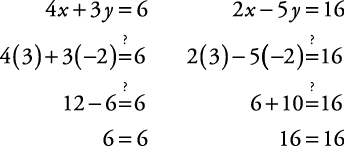## Linear Equations: Solutions Using Graphing with Two Variables

##### Example 1

Solve this system of equations by graphing.To solve using graphing, graph both equations on the same set of coordinate axes and see where the graphs cross. The ordered pair at the point of intersection becomes the solution (see Figure 1).

Check the solution.The solution is x = 3, y = –2.

Back to Top
A18ACD436D5A3997E3DA2573E3FD792A